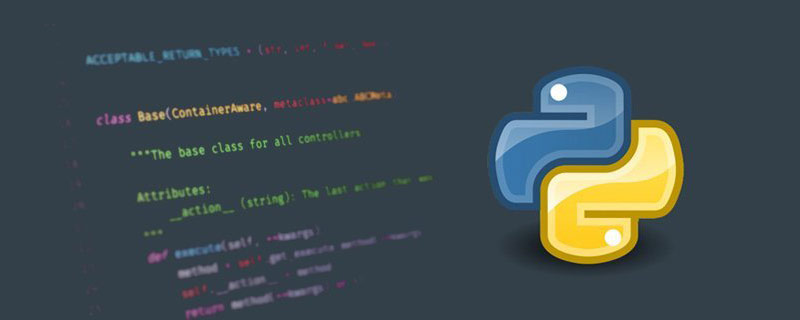# python字符串是如何存储的• FXL   2020-08-07 09:17:52927

python内部是通过享元模式存储字符串的。享元模式是为了降低存储空间而做的一种优化手段。字符串内容相同的两个字符串指向的是同一个对象，这正是享元模式所做的。python内部存储字符串采用的是享元模式（Flyweight pattern）。

（推荐教程：Python入门教程

==是用来推断两个东西是否相等，比方：

```a = 10。
b = 10；
print（a == b）；```

```a = [1,2,3];
b = [1,2,3];
c = [1,2,4];
print(a==b);
print(a==c);```

```x = [1,2,3];
y = [1,2,3];
print(x is y);```

```x = [1,2];
y = x;
print(x is y);```

```x = “huan”;
y = x;
print(x == y);
print(x is y);```

```true
true```

```x = “huan”;
y = “huan”;
print(x == y);
print(x is y);```

```true
true```